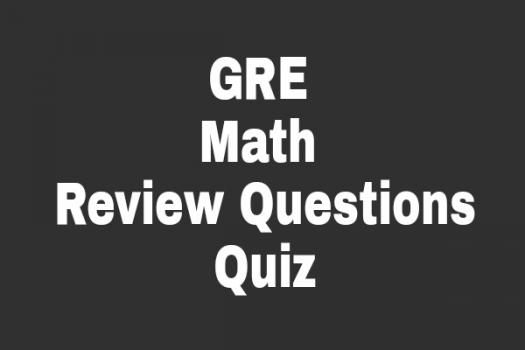# GRE Math Review Questions

10 Questions | Total Attempts: 119SettingsThis quiz presents you simple mathematical problems to revise basic math laws. With these simple questions, get to know how much of mathematical knowledge you know. Would you give it a nice try? Get detailed explanation for the right or wrong answers after completion as you prepare for the GRE Math Exam.

Related Topics
• 1.
What is the half of a dozen?
• A.

10

• B.

20

• C.

6

• D.

5

• 2.
What is a score equal to?
• A.

25

• B.

30

• C.

35

• D.

20

• 3.
How many years make a century?
• A.

60 years

• B.

150 years

• C.

70 years

• D.

100 years

• 4.
What is gross equal to?
• A.

206

• B.

144

• C.

180

• D.

120

• 5.
What is the half of a quarter?
• A.

1/8

• B.

1/8

• C.

1/4

• D.

1/2

• 6.
What is the product of 8 and 12?
• A.

128

• B.

120

• C.

812

• D.

96

• 7.
What is the square root of 144?
• A.

9

• B.

6

• C.

20

• D.

12

• 8.
Which of these numbers is not even?
• A.

27

• B.

22

• C.

100

• D.

2

• 9.
Which of these numbers is a prime number?
• A.

10

• B.

21

• C.

9

• D.

5

• 10.
What is the sum of 1/2 and 1/4
• A.

2/6

• B.

2/4

• C.

3/8

• D.

3/4